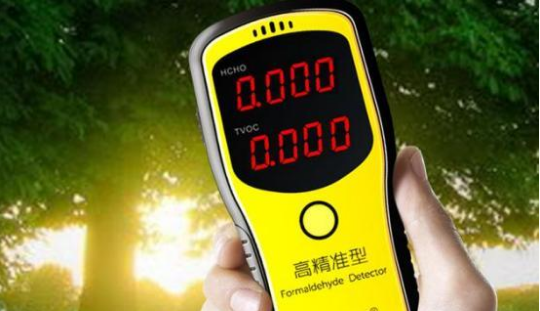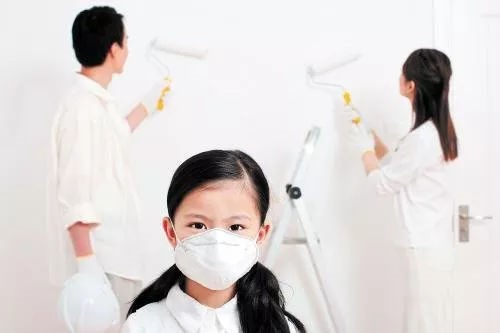|

# 连甲醛测量仪都是假的,害怕甲醛的我该何去何从?

这几天全国正处于“两会时间”，今年两会，呼吸问题再次被代表们所关注，而这次被关注的焦点是“甲醛房”，还有装修污染。有代表就表示：“租住或者新装修的房屋中有甲醛超标情况，一定要重视，应及时采取通风或净化等方式来处理。”

现在家庭装修，甲醛是一个绕不过去的坎。人们一谈到“甲醛超标”都会不寒而栗，已经达到“闻甲醛而色变”的程度。为了保险，很多年轻人装修完新家，搬家之前都会上网买个“甲醛检测仪”，拿着它满屋的晃悠，盯着上面的数值，直到数值满意了才开始搬家入住。但是，网上卖的那些设备就一定测得准么？上个月，央视的《每周质量报告》就对电商网站上卖的甲醛测量仪进行了检测，检测结果表明这些网红检测仪对甲醛检测的数据误差高到离谱，可以说网上很多甲醛仪检测仪显示的甲醛浓度数值连参考价值都没有。我国室内污染问题日益严峻，装修污染已经成为健康的隐形杀手。据中国室内环境监测中心调查结果显示：我国每年由于室内空气污染引起的急诊数为430万人次；每年由室内污染造成的死亡人数达11.1万人，平均每天死亡304人；每年新增先天残疾儿童总数高达80万至120万，其中，42.1%与室内空气污染有关，其中甲醛是主要元凶。

尽管近年来社会各界对于家居绿色环保的重视程度逐渐提高，但我国家居产品"不绿色、不环保"的问题依然存在，因甲醛患病、危机生命的新闻层出不穷。例如， “江西一幼儿园50多个孩子流鼻血，园方拒做甲醛检测。”、“上百学生流鼻血，呕吐，学校“毒跑道”事件”等。可谓是“一石激起千层浪”， 引发了消费者，尤其是一二线城市80、90后在内的中产阶层消费者对甲醛危害的高度关注。其实早在2004年，国际癌症研究机构（IACR）在研究报告中明确表明甲醛对人类有致癌作用，流行病学上可导致白血病。作为家居行业的新主力80、90后，收入水平、教育水平、消费水平高，接受信息面广，对环保了解透彻。同时，这一群体也已到了生儿育女的年龄，对“环保”要求更为苛刻，对“甲醛”字眼更为敏感。相较于“防”、“治”室内空气污染，他们更愿意购买“零甲醛”添加产品。这批人群对家居的消费观念也已发生质的转变，由“被动推销”转变为“主动出击”，主动咨询环保、零甲醛等相关内容。也有研究表明，超过97%的消费者在选购家居产品时会考虑绿色健康因素对身体的影响。

国家从去年５月１日新的国家标准ＧＢ１８５８０－２０１７《室内装饰装修材料人造板及其制品中甲醛释放量》开始正式实施，其中只保留Ｅ１级，取消了原标准的Ｅ２级，提高了甲醛释放限量要求。同年12月29日由全国人造板标准化技术委员会组织申报的《人造板及其制品甲醛释放量分级》推荐性国家标准制定计划获批立项，进一步细化人造板及其制品甲醛限量分级。该标准制定将为无甲醛添加人造板（包括秸秆人造板）提供标准支持，积极推动低甲醛、无甲醛添加产品生产和促进人造板及其制品行业的绿色发展。

基于此，网易家居与万华禾香板编制《中国室内绿色居住安全白皮书（2019）》，通过对大家居行业，消费者，家居品牌商，经销商，板材生产商等角色的深入调研，剖析家庭居住的安全困境、指明行业发展瓶颈、倡导健康环保的未来计划，守护更多人的居住安全。

作为市场上的无醛添加板材企业，万华禾香板作为绿色大家居产业的开拓者，20年来致力于推动绿色健康环保产业的发展。采用稻草、麦草、芦苇、果树枝条等农作物剩余物为主要原料，以完全不含甲醛的聚氨酯生态粘合剂，制成无醛添加人造板材。历时12年，让“吃木头”的行业变为“吃草”行业；2018年，万华禾香板发布了集板材、饰面、家具三大制造体系资源聚于一体的分布式绿色家居产业集群模式，实现了就近取材，就近智造，就近服务。同时，万华公益在行动，与北京新阳光慈善基金建立长期友好的合作，致力于儿童白血病的教育和关怀。为更多家庭营造绿色、健康的生活环境，万华禾香板责无旁贷。

3月27日，网易家居联合万华禾香板，共同发布《中国室内绿色居住安全白皮书（2019）》，请大家拭目以待。

`声明：本文由入驻焦点开放平台的作者撰写，除焦点官方账号外，观点仅代表作者本人，不代表焦点立场错误信息举报电话： 400-099-0099，邮箱：jubao@vip.sohu.com，或点此进行意见反馈，或点此进行举报投诉。`A B C D E F G H J K L M N P Q R S T W X Y Z
A - B - C - D - E
• A
• 鞍山
• 安庆
• 安阳
• 安顺
• 安康
• 澳门
• B
• 北京
• 保定
• 包头
• 巴彦淖尔
• 本溪
• 蚌埠
• 亳州
• 滨州
• 北海
• 百色
• 巴中
• 毕节
• 保山
• 宝鸡
• 白银
• 巴州
• C
• 承德
• 沧州
• 长治
• 赤峰
• 朝阳
• 长春
• 常州
• 滁州
• 池州
• 长沙
• 常德
• 郴州
• 潮州
• 崇左
• 重庆
• 成都
• 楚雄
• 昌都
• 慈溪
• 常熟
• D
• 大同
• 大连
• 丹东
• 大庆
• 东营
• 德州
• 东莞
• 德阳
• 达州
• 大理
• 德宏
• 定西
• 儋州
• 东平
• E
• 鄂尔多斯
• 鄂州
• 恩施
F - G - H - I - J
• F
• 抚顺
• 阜新
• 阜阳
• 福州
• 抚州
• 佛山
• 防城港
• G
• 赣州
• 广州
• 桂林
• 贵港
• 广元
• 广安
• 贵阳
• 固原
• H
• 邯郸
• 衡水
• 呼和浩特
• 呼伦贝尔
• 葫芦岛
• 哈尔滨
• 黑河
• 淮安
• 杭州
• 湖州
• 合肥
• 淮南
• 淮北
• 黄山
• 菏泽
• 鹤壁
• 黄石
• 黄冈
• 衡阳
• 怀化
• 惠州
• 河源
• 贺州
• 河池
• 海口
• 红河
• 汉中
• 海东
• I
• J
• 晋中
• 锦州
• 吉林
• 鸡西
• 佳木斯
• 嘉兴
• 金华
• 景德镇
• 九江
• 吉安
• 济南
• 济宁
• 焦作
• 荆门
• 荆州
• 江门
• 揭阳
• 金昌
• 酒泉
• 嘉峪关
K - L - M - N - P
• K
• 开封
• 昆明
• 昆山
• L
• 廊坊
• 临汾
• 辽阳
• 连云港
• 丽水
• 六安
• 龙岩
• 莱芜
• 临沂
• 聊城
• 洛阳
• 漯河
• 娄底
• 柳州
• 来宾
• 泸州
• 乐山
• 六盘水
• 丽江
• 临沧
• 拉萨
• 林芝
• 兰州
• 陇南
• M
• 牡丹江
• 马鞍山
• 茂名
• 梅州
• 绵阳
• 眉山
• N
• 南京
• 南通
• 宁波
• 南平
• 宁德
• 南昌
• 南阳
• 南宁
• 内江
• 南充
• P
• 盘锦
• 莆田
• 平顶山
• 濮阳
• 攀枝花
• 普洱
• 平凉
Q - R - S - T - W
• Q
• 秦皇岛
• 齐齐哈尔
• 衢州
• 泉州
• 青岛
• 清远
• 钦州
• 黔南
• 曲靖
• 庆阳
• R
• 日照
• 日喀则
• S
• 石家庄
• 沈阳
• 双鸭山
• 绥化
• 上海
• 苏州
• 宿迁
• 绍兴
• 宿州
• 三明
• 上饶
• 三门峡
• 商丘
• 十堰
• 随州
• 邵阳
• 韶关
• 深圳
• 汕头
• 汕尾
• 三亚
• 三沙
• 遂宁
• 山南
• 商洛
• 石嘴山
• T
• 天津
• 唐山
• 太原
• 通辽
• 铁岭
• 泰州
• 台州
• 铜陵
• 泰安
• 铜仁
• 铜川
• 天水
• 天门
• W
• 乌海
• 乌兰察布
• 无锡
• 温州
• 芜湖
• 潍坊
• 威海
• 武汉
• 梧州
• 渭南
• 武威
• 吴忠
• 乌鲁木齐
X - Y - Z
• X
• 邢台
• 徐州
• 宣城
• 厦门
• 新乡
• 许昌
• 信阳
• 襄阳
• 孝感
• 咸宁
• 湘潭
• 湘西
• 西双版纳
• 西安
• 咸阳
• 西宁
• 仙桃
• 西昌
• Y
• 运城
• 营口
• 盐城
• 扬州
• 鹰潭
• 宜春
• 烟台
• 宜昌
• 岳阳
• 益阳
• 永州
• 阳江
• 云浮
• 玉林
• 宜宾
• 雅安
• 玉溪
• 延安
• 榆林
• 银川
• Z
• 张家口
• 镇江
• 舟山
• 漳州
• 淄博
• 枣庄
• 郑州
• 周口
• 驻马店
• 株洲
• 张家界
• 珠海
• 湛江
• 肇庆
• 中山
• 自贡
• 资阳
• 遵义
• 昭通
• 张掖
• 中卫

1室1厅1厨1卫1阳台

1
2
3
4
5

0
1
2

1

1

0
1
2
3报名成功，资料已提交审核A B C D E F G H J K L M N P Q R S T W X Y Z
A - B - C - D - E
• A
• 鞍山
• 安庆
• 安阳
• 安顺
• 安康
• 澳门
• B
• 北京
• 保定
• 包头
• 巴彦淖尔
• 本溪
• 蚌埠
• 亳州
• 滨州
• 北海
• 百色
• 巴中
• 毕节
• 保山
• 宝鸡
• 白银
• 巴州
• C
• 承德
• 沧州
• 长治
• 赤峰
• 朝阳
• 长春
• 常州
• 滁州
• 池州
• 长沙
• 常德
• 郴州
• 潮州
• 崇左
• 重庆
• 成都
• 楚雄
• 昌都
• 慈溪
• 常熟
• D
• 大同
• 大连
• 丹东
• 大庆
• 东营
• 德州
• 东莞
• 德阳
• 达州
• 大理
• 德宏
• 定西
• 儋州
• 东平
• E
• 鄂尔多斯
• 鄂州
• 恩施
F - G - H - I - J
• F
• 抚顺
• 阜新
• 阜阳
• 福州
• 抚州
• 佛山
• 防城港
• G
• 赣州
• 广州
• 桂林
• 贵港
• 广元
• 广安
• 贵阳
• 固原
• H
• 邯郸
• 衡水
• 呼和浩特
• 呼伦贝尔
• 葫芦岛
• 哈尔滨
• 黑河
• 淮安
• 杭州
• 湖州
• 合肥
• 淮南
• 淮北
• 黄山
• 菏泽
• 鹤壁
• 黄石
• 黄冈
• 衡阳
• 怀化
• 惠州
• 河源
• 贺州
• 河池
• 海口
• 红河
• 汉中
• 海东
• I
• J
• 晋中
• 锦州
• 吉林
• 鸡西
• 佳木斯
• 嘉兴
• 金华
• 景德镇
• 九江
• 吉安
• 济南
• 济宁
• 焦作
• 荆门
• 荆州
• 江门
• 揭阳
• 金昌
• 酒泉
• 嘉峪关
K - L - M - N - P
• K
• 开封
• 昆明
• 昆山
• L
• 廊坊
• 临汾
• 辽阳
• 连云港
• 丽水
• 六安
• 龙岩
• 莱芜
• 临沂
• 聊城
• 洛阳
• 漯河
• 娄底
• 柳州
• 来宾
• 泸州
• 乐山
• 六盘水
• 丽江
• 临沧
• 拉萨
• 林芝
• 兰州
• 陇南
• M
• 牡丹江
• 马鞍山
• 茂名
• 梅州
• 绵阳
• 眉山
• N
• 南京
• 南通
• 宁波
• 南平
• 宁德
• 南昌
• 南阳
• 南宁
• 内江
• 南充
• P
• 盘锦
• 莆田
• 平顶山
• 濮阳
• 攀枝花
• 普洱
• 平凉
Q - R - S - T - W
• Q
• 秦皇岛
• 齐齐哈尔
• 衢州
• 泉州
• 青岛
• 清远
• 钦州
• 黔南
• 曲靖
• 庆阳
• R
• 日照
• 日喀则
• S
• 石家庄
• 沈阳
• 双鸭山
• 绥化
• 上海
• 苏州
• 宿迁
• 绍兴
• 宿州
• 三明
• 上饶
• 三门峡
• 商丘
• 十堰
• 随州
• 邵阳
• 韶关
• 深圳
• 汕头
• 汕尾
• 三亚
• 三沙
• 遂宁
• 山南
• 商洛
• 石嘴山
• T
• 天津
• 唐山
• 太原
• 通辽
• 铁岭
• 泰州
• 台州
• 铜陵
• 泰安
• 铜仁
• 铜川
• 天水
• 天门
• W
• 乌海
• 乌兰察布
• 无锡
• 温州
• 芜湖
• 潍坊
• 威海
• 武汉
• 梧州
• 渭南
• 武威
• 吴忠
• 乌鲁木齐
X - Y - Z
• X
• 邢台
• 徐州
• 宣城
• 厦门
• 新乡
• 许昌
• 信阳
• 襄阳
• 孝感
• 咸宁
• 湘潭
• 湘西
• 西双版纳
• 西安
• 咸阳
• 西宁
• 仙桃
• 西昌
• Y
• 运城
• 营口
• 盐城
• 扬州
• 鹰潭
• 宜春
• 烟台
• 宜昌
• 岳阳
• 益阳
• 永州
• 阳江
• 云浮
• 玉林
• 宜宾
• 雅安
• 玉溪
• 延安
• 榆林
• 银川
• Z
• 张家口
• 镇江
• 舟山
• 漳州
• 淄博
• 枣庄
• 郑州
• 周口
• 驻马店
• 株洲
• 张家界
• 珠海
• 湛江
• 肇庆
• 中山
• 自贡
• 资阳
• 遵义
• 昭通
• 张掖
• 中卫• 手机• 分享
• 设计
免费设计
• 计算器
装修计算器
• 入驻
合作入驻
• 联系
联系我们
• 置顶
返回顶部